Courses

# Venn Diagrams and Operations on Sets Mathematics Notes | EduRev

## Algebra for IIT JAM Mathematics

Created by: Veda Institute

## Mathematics : Venn Diagrams and Operations on Sets Mathematics Notes | EduRev

The document Venn Diagrams and Operations on Sets Mathematics Notes | EduRev is a part of the Mathematics Course Algebra for IIT JAM Mathematics.
All you need of Mathematics at this link: Mathematics

Venn Diagrams
A Venn diagram is a diagrammatic representation of ALL the possible relationships between different sets of a finite number of elements. Venn diagrams were conceived around 1880 by John Venn, an English logician, and philosopher. They are extensively used to teach Set Theory. A Venn diagram is also known as a Primary diagram, Set diagram or Logic diagram.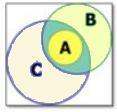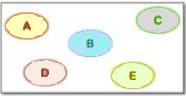Representation of Sets in a Venn Diagram
It is done as per the following:

• Each individual set is represented mostly by a circle and enclosed within a quadrilateral (the quadrilateral represents the finiteness of the Venn diagram as well as the Universal set.)
• Labelling is done for each set with the set’s name to indicate difference and the respective constituting elements of each set are written within the circles.
• Sets having no element in common are represented separately while those having some of the elements common within them are shown with overlapping.
• The elements are written within the circle representing the set containing them and the common elements are written in the parts of circles that are overlapped.

Operations on Venn Diagrams
Just like the mathematical operations on sets like Union, Difference, Intersection, Complement, etc. we have operations on Venn diagrams that are given as follows:

Union of Sets
Let A = {2, 4, 6, 8} and B = {6, 8, 10, 12}. Represent A U B through a well-labeled Venn diagram.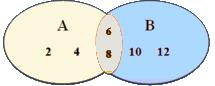The orange colored patch represents the common elements {6, 8} and the quadrilateral represents A U B.

Properties of A U B

• The commutative law holds true as A U B = B U A
• The associative law also holds true as (A U B) U C = A U (B U C)
• A U φ = A (Law of identity element)
• Idempotent Law – A U A = A
• Law of the Universal Set U – A U U = U

The Intersection of Sets

An intersection is nothing but the collection of all the elements that are common to all the sets under consideration. Let A = {2, 4, 6, 8} and B = {6, 8, 10, 12} then A ∩ B is represented through a Venn diagram as per following:The orange colored patch represents the common elements {6, 8} as well as the A ∩ B. The intersection of 2 or more sets is the overlapped part(s) of the individual circles with the elements written in the overlapped parts. Example: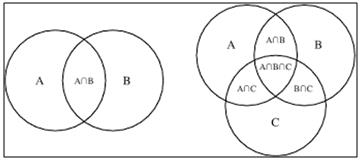Properties of A ∩ B

• Commutative law – A ∩ B = B∩ A
• Associative law – (A ∩ B)∩ C = A ∩ (B∩ C)
• φ ∩ A = φ
• U ∩ A = A
• A∩ A = A; Idempotent law.
• Distributive law – A ∩ (B∩ C) = (A ∩ B) U(A ∩ C)

Difference of Sets
The difference of set A and B is represented as: A – B = {x: x ϵ A and x ϵ B} {converse holds true for B – A}. Let, A = {1, 2, 3, 4, 5, 6} and B = {2, 4, 6, 8} then A – B = {1, 3, 5} and B – A = {8}. The sets (A – B), (B – A) and (A ∩ B) are mutually disjoint sets.
It means that there is NO element common to any of the three sets and the intersection of any of the two or all the three sets will result in a null or void or empty set. A – B and B – A are represented through Venn diagrams as follows: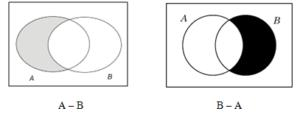Complement of Sets

If U represents the Universal set and any set A is the subset of A then the complement of set A (represented as A’) will contain ALL the elements which belong to the Universal set U but NOT to set A.

Mathematically – A’ = U – A
Alternatively, the complement of a set A, A’ is the difference between the universal set U and the set A. Example: Let universal set U = {1, 2, 3, 4, 5, 6, 7, 8, 9, 10} and set A = {1, 3, 5, 7, 9}, then complement of A is given as: A’ = U – A = {2, 4, 6, 8, 10}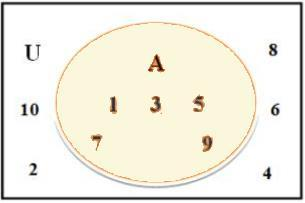Properties Of Complement Sets
A U A’ = U
A ∩ A’ = φ
De Morgan’s Law – (A U B)’ = A’ ∩ B’ OR (A ∩ B)’ = A’ U B’
Law of double complementation : (A’)’ = A
φ’ = U
U’ = φ

Solved Examples For You

Ques 1: Represent the Universal Set (U) = {x : x is an outcome of a dice’s roll} and set A = {s : s ϵ Even numbers} through a Venn diagram.

Solution: Since, U = {1, 2, 3, 4, 5, 6} and A = {2, 4, 6}. Representing this with a Venn diagram we have: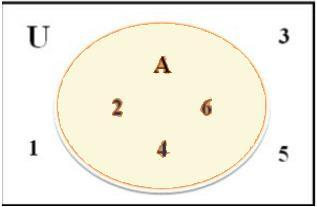Here, A is a subset of U, represented as – A ⊂ U or
U is the superset of A, represented as – U⊃ A
If A = {1, 2, 3, 4, 5} and B = {4, 5, 6, 7, 8},
then represent A – B and B – A through Venn diagrams.
A – B = {1, 2, 3}
B – A = {6, 7, 8}
Representing them in Venn diagrams:

a. A-B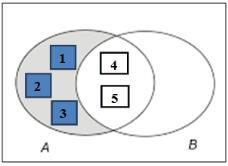b. B-A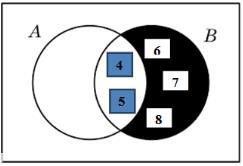Operations on sets :
When two or more sets are combined together to form another set under some given conditions, then operations on sets are carried out.
The important operations on sets are.
Union
Let X and Y be two sets.
Now, we can define the following new set.
X u Y  =  {z | z ∈ X or z ∈ Y}
(That is, z may be in X or in Y or in both X and Y)
X u Y is read as "X union Y"
Now that X u Y contains all the elements of X and all the elements of Y and the figure given below illustrates this.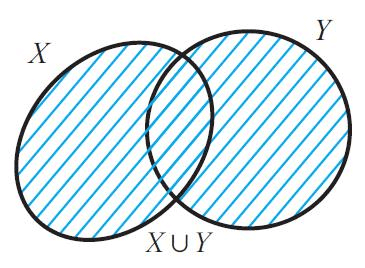It is clear that X ⊆ X u Y and also Y ⊆ X u Y
Intersection
Let X and Y be two sets.
Now, we can define the following new set.
X n Y  =  {z | z ∈ X and z ∈ Y}
(That is z must be in  both X and Y)
X n Y is read as "X intersection Y"
Now that X n Y contains only those elements which belong to both X and Y and the figure given below illustrates this.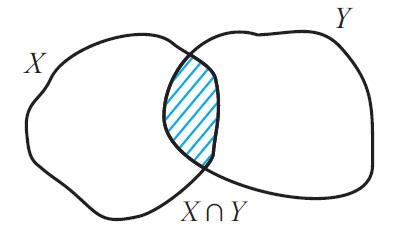It is trivial that that X n Y ⊆ X and also X n Y ⊆ Y
Set difference
Let X and Y be two sets.
Now, we can define the following new set.
X \ Y  =  {z | z ∈ X but z ∉  Y}
(That is z must be in  X and must not be in Y)
X \ Y is read as "X difference Y"
Now that X \ Y contains only elements of X which are not in Y and the figure given below illustrates this.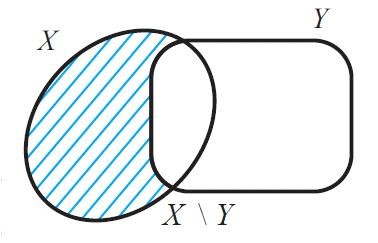Some authors use A - B for A \ B. We shall use the notation A \ B which is widely used in mathematics for set difference.
Symmetric difference
Let X and Y be two sets.
Now, we can define the following new set.
X Δ  Y  =  (X \ Y) u (Y \ X)
Δ Y is read as "X symmetric difference Y"
Now that X Δ Y contains all elements in X u Y which are not in X n Y and the figure given below illustrates this. .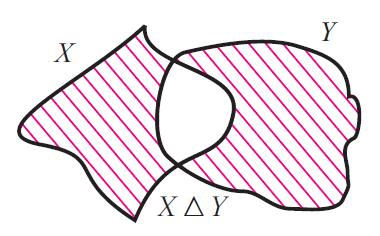Complement
If X ⊆ U, where U is a universal set, then U \ X is called the compliment of X with respect to U.
If underlying universal set is fixed, then we denote U \ X by X' and it is called compliment of X.
X'  =  U \ X The difference set set A \ B can also be viewed as the compliment of B with respect to A.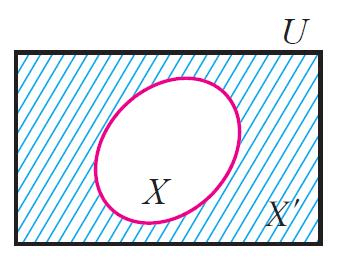Disjoint sets
Two sets X and Y are said to be disjoint if they do not have any common element. That is,
X and Y are disjoint if X n Y = ᵩ
It is clear that n(A u B) = n(A) + n(B), if A and B are disjoint finite set.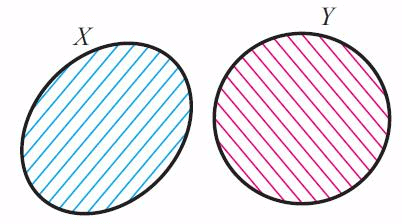Offer running on EduRev: Apply code STAYHOME200 to get INR 200 off on our premium plan EduRev Infinity!

,

,

,

,

,

,

,

,

,

,

,

,

,

,

,

,

,

,

,

,

,

;